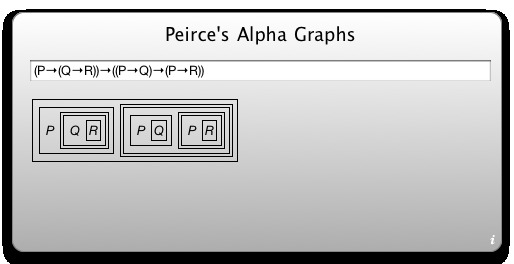# Dashboard Widget

## Overview# Alpha Graph Widget## The Widget-in-itself

The Alpha Graph Widget happens to be called Alpha Graph Widget due to its sole purpose being the generation, and proper display, of alpha graphs, the propositional subset of Charles Sanders Peirce's Existential Graphs. The widget works with MacOS 10.4.3 and later. It is small and does not require a live connection to the Internet.

Alpha Graph Widget, being so called due to it being a widget displaying alpha graphs, supports all usual connectives of classical logic, that is negation, conjunction, (inclusive) disjunction, conditional (material implication), biconditional (material equivalence), exclusive disjuncton (XOR), the Peirce operator (NOR), the Sheffer operator (NAND), as well as the constants 1 and 0 denoting truth and falsehood, respectively.

## Input examples

Infix notation Polish notation
P->(Q->P) CpCqp
(P->Q)->P CCpqp
P->Q->P CCpqp
~P v Q ANpq
P & Q v P & R AKpqKpr
(P & Q) v (P & R) AKpqKpr
P & (Q v P) & R KKpAqpr
((P>(Q>R))>((P>Q)>(P>R))) CCpCqrCCpqCpr
P>(Q>R)>(P>Q>(P>R)) CCpCqrCCpqCpr
P!PNApp
(P!Q)!(P!Q)NANApqNApq
(P!P)!(Q!Q)NANAppNAqq

## Usage

The user interface of the Alpha Graph Widget consists of a sole text field where the user may enter a single proposition, be it in infix notation (the somewhat standard way of writing down propositions), or in Polish notation (the cool prefix notation developed by Lukasiewicz in the 1920s). After entering the proposition, all the user needs to do is depressing the Enter key smoothly, but firmly. No more than one or two blinks of an eye later, an alpha graph gets displayed properly.

### Infix notation

Note: Your browser may be unable to properly display some of the logical connectives used below. Of course this restriction will not apply to the alpha graph widget when installed and running on your personal computing device.

Propositional constants
A through U, and W through Z, case insensitive; note that the letter "v" is reserved for disjunction (see below) and may not be used for a propositional constant.
Negation
~ (tilde), - (dash), ¬
Conjunction
& (ampersand), ^, ∧
Disjunction
v (the lower-case letter), |, ∨
Conditional
>, ->, =>, -->, ==>, →
Biconditional
=, ↔
XOR
%
Peirce operator (NOR)
! (exclamation mark), ↓
Sheffer operator (NAND)
upside-down exlamation mark, ↑
Brackets
As usual, the rounded brackets, (, and ), may be used for grouping expressions.
Spaces
In order to improve readability, the user may freely add space characters into their proposition.
Operator precedence
The precedence of the operators is the following: (1) Negation (first); (2) conjunction and NAND; (3) disjunction, XOR, and NOR; (4) conditional; and (5) biconditional (last). Operators of the same precedence that are not grouped with brackets will be evaluated from left to right.

### Polish Notation

Propositions in Polish notation are restricted to Lukasiewicz's own connectives N, K, A, C, and E. You have to user lower-case letters as propositional constants, but not the letter "v", since it denotes infix disjunction (see above).

## Alpha graphs: an extremely short introduction

For one thing (and as far as it concerns this program), alpha graphs are a graphical notation for propositional logic. In this respect, alpha graphs use only two connectives: conjunction and negation. Since all classical truth functions can be expressed using the truth functions of classical conjunction and negation, it is effectively possible to express any expression of classical propositional logic by an alpha graph.

• For expressing the conjunction of two propositions, you simply write both of them down. For example, "PQ" expresses the conjunction of the two atomic propositions "P", and "Q".
• To negate a proposition, you enclose it with a line building up e.g. a circle, an oval, a square, or indeed any closed geometrical figure you like (for technical reasons, this program uses rectangles). A variant proposed by Peirce himself is bracketing: Here you put the proposition to negate between brackets, making the brackets "simulate" a closed figure. So, if you want to negate "P", you write down "P" and draw e.g. a circle around it - or you write down "(P)".
• For the conditional "If P, then Q", Peirce uses the diagram corresponding to "(P(Q))", literally meaning "It is not the case that both P is true, but Q is false". Note that, indeed, P→Q is equivalent to ¬(P&¬Q).
• The alternative disjunction "P, or Q, or both" is written as "((P)(Q))" (or, rather, drawn as the corresponding diagram), literally meaning "It is not the case that both P, and Q, is false".

Though this short introduction should suffice to provide an understanding of the alpha graphs as a logical notation (and, hence, of the Alpha Graph Program), it does justice neither to Peirce's motivation (both philosophical and didactical), nor to the formal aspects of his graphical logic, since, for the other thing, what Peirce did was a formally complete graphical calculus. For an overview of these aspects, you might see my German Wikipedia article Existential Graphs. At the time of writing this, the article in the English Wikipedia is shorter and lacks depth, but provides both a first overview, and a number of references.